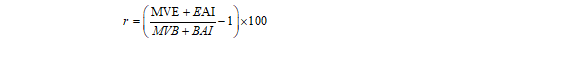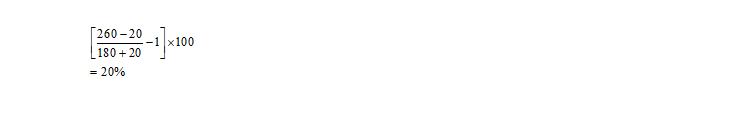In my last piece, I talked about why you should measure the performance of your investments. I also promised to talk about how you can calculate the performance measurement metrics needed to appraise your investments.

The good thing about investment performance measurement is that it works across asset classes and strategies. It can be used for fixed income securities as much as it can be used for equities or mutual funds.

## Asset Valuation

Investment performance measurement begins with the valuation of the assets within a portfolio. But as an investor, investing through a fund manager, the responsibility of valuing the assets within your portfolio shifts to the fund manager. For the “do it yourself” (DIY) investor, the valuation of the asset within your portfolio will derive from the market prices of those assets.

For example, if you have a portfolio that holds 100 shares of Access Bank, 250 shares of Dangote Cement and 50 shares of Nestle, the value of your portfolio will be the sum (sum-product) of the number of shares in each equity multiplied by the price as at the date of the valuation.

## What is Return and how is it calculated

The starting point for performance measurement is the calculation of return. Return is the benefit you received from an investment over a period of time. Return or gain is the difference between the current value of your investment and the original investment or the value as at the last calculation period.

Gain or Loss= Current Value-Investment Made

Rate of return is the gain or loss expressed as a percentage of the original investment or prior investment valueFor example, assuming you invested N200 in ARM Aggressive fund in January, and by January 31, you received your investment statement that says that your investment in ARM Aggressive fund was N260, it means that your return or gain or benefit for the month of January was N60, calculated as N260 less N200. The rate of return, therefore, is 60 divided by 200, that is .3 which, when multiplied by 100, gives you a rate of return of 30%.

Speaking the language of the market, the rate of return can be calculated as the difference between the ending market value and the beginning market value divided by the beginning market value. This difference accounts for changes in the price or value of the portfolio or asset over a period of time, otherwise called unrealized gain or loss.

Mathematically speaking, the numerator of the rate of return calculation is the unrealized gain or loss, that is the difference between the ending market value and the beginning market value. The denominator is the money you invested (or the money at risk) or principal amount. It is good to note that the principal amount invested is used only in the first period, but in subsequent periods, the denominator becomes the ending market value in the previous period.

[READ FURTHER: 9 TIPS to KNOW when NOT to INVEST]

For example, if you are measuring the rate of return for June, having measured that of May, the denominator becomes your May ending market value. In most cases, the market value of investments account for changes in the prices of the investments, giving you the unrealized gains or losses, it does not capture the dividends or interests that have been paid or accrued (accumulated) on the portfolio or asset.Therefore, to get a more realistic calculation of the rate of return, you have to factor in the dividends or interests. In that case, the rate of return will be ending market value plus ending accrued income divided by beginning market value. This is what they call total return, in investment language.In the above, MVE represents ending market value while EAI represents ending accrued income, while MVB represents beginning market value, which is actually the ending value of the previous period. For example, suppose you have invested N20,000 in a carefully-chosen investment portfolio. Exactly 1 year from the time of initial investment, the investment portfolio gives you N200 of cash dividends, and the investment portfolio has a market value of N22,000 at the end of the current period, what is your rate of return?

Your rate of return is R  is (200 + 22,000  /20,000)-1

= 11%

[KEEP READING: How To Use Return On Assets As A Great Investment Tool]

Effects of Income Reinvestment: Investment performance measurement gets more complicated and more complex when incomes or dividends get reinvested because when that happens, not only do the income or dividends form part of the numerator in the calculation, they become part of the denominator as well. In that case, the rate of return becomes;Where BAI represents beginning accrued income, in addition to the other variables already defined earlier. For example, suppose you have invested N20,000 in a carefully-chosen investment portfolio. Exactly 1 year from the time of initial investment, the investment portfolio gives you N200 of cash dividends which you reinvested in the portfolio, and the investment portfolio has a market value of N22,000 at the end of the current period, what is your rate of return?

Your rate of return is  (200 + 22,000  /20,000+200)-1

= 9.9%

## Effects of Cashflows

It is not only the reinvestment of dividends or interests that complicate the calculation of investment performance or rate of return. It even gets further more complex and complicated when you have additional investments or subscriptions and when you partially redeem or sale some of your investments. In investment performance measurement, those activities are called cashflows.

So, the cashflows affect investment performance measurement because you need to account for them, not only due to their numerical effect but also due to their timing effect. The effect of a redemption done at the beginning of the month differs from the effect of such redemption done in the middle of the month for investment performance measurement purposes. When there are cash flows, gain or loss is calculated as follows:In that case, the rate of return becomesFor example, assume your investment had a beginning MV of N180.00 in a fund and you invested N20 at the beginning of the year and by the end of the year your investor statement says that the market value of your investment is N260.00, what is your rate of return? Your rate of return will be 20% calculated as

[YOU MAY LIKE: Businessman makes a strong case against equity investment]

## Timing of Cash flows

One drawback of basic return on investment calculation (ROI) is that it does not account for the timing of cash flows.  Returns differ depending on whether cash inflows happen at the end or beginning of the period. Returns are higher when cash inflows happen at the end of the period.

Returns are lower when cash inflows happen at the beginning of the period. For example, assume your investment had a beginning MV of N180.00 in a fund and you invested N20 at the end of the year and by the end of the year, your investor statement says that the market value of your investment is N260.00. What is your rate of return?

The rate of return will be 33.33% calculated asBecause the contribution was made at the end, it was not part of the value risked and because the contribution was made at the end, the rate of return was higher than previously calculated.

## More and More Complications Abide

There are a lot more complications and complexities in investment performance calculation as you get deeper and more granular, as you try to calculate time-weighted and money-weighted returns and even dive into the areas that angels in investment performance calculation dread to walk, the area of attribution analysis. I will, therefore, end this piece here, without introducing further complications. Those who need help in measuring the performance of their investments and for corporate organizations that want to expose their staff to a more robust understanding of investment performance measurement, they can get in touch with me.[READ THIS: How To Use Return On Assets As A Great Investment Tool]

This site uses Akismet to reduce spam. Learn how your comment data is processed.